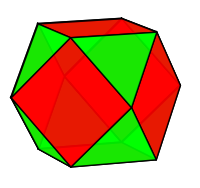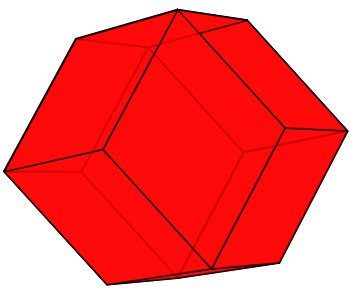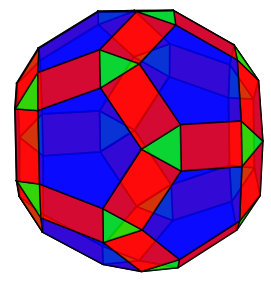Computer Science

# Computational Geometry: Level 4 ChallengesWhat is the shortest distance between any two points in the following set of points? (Excluding distances between the same points. )

Sally loves apples, thus, she has an apple garden in her backyard, where she has grown $25$ apples in the shape of a square of side $5$. She is standing in the center of the garden, i.e. $3^{\text{rd}}$ row and $3^{\text{rd}}$ column. Now, she wants to move through the garden eating apple in every square, and she won't move into square without any apple. How many ways are there in which she can eat all the $25$ apples?

Example:

If it were a $3\times 3$ garden, and Sally would have started from center, i.e. ($2,2)$ there would be $8$ solutions as follows

$1.\ (2,2),(3,2),(3,3),(2,3),(1,3),(1,2),(1,1),(2,1),(3,1)$ $2.\ (2,2),(3,2),(3,1),(2,1),(1,1),(1,2),(1,3),(2,3),(3,3)$ $3.\ (2,2),(2,3),(3,3),(3,2),(3,1),(2,1),(1,1),(1,2),(1,3)$ $4.\ (2,2),(2,3),(1,3),(1,2),(1,1),(2,1),(3,1),(3,2),(3,3)$ $5.\ (2,2),(1,2),(1,3),(2,3),(3,3),(3,2),(3,1),(2,1),(1,1)$ $6.\ (2,2),(1,2),(1,1),(2,1),(3,1),(3,2),(3,3),(2,3),(1,3)$ $7.\ (2,2),(2,1),(1,1),(3,2),(3,3),(2,3),(1,3),(1,2),(1,1)$ $8.\ (2,2),(2,1),(1,1),(1,2),(1,3),(2,3),(3,3),(3,2),(3,1)$

Let $P$ be a polyhedron that is the convex hull of the vertices $v_1, v_2, \ldots , v_n$ and with edges $e_1, e_2, \ldots , e_m$ where each edge is defined to be the set of points on the line that passes through some two distinct vertices $v_i, v_j$.

We define the Edge-Plosion of $P$, $E!( P )$, to be the convex hull of the mid-points of each edge of $P$, where $P$ is a cube.

Which of these is an image of $E!(E!(P))$?

(A):(B):(C):(D):×